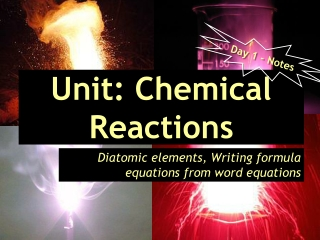DownloadDownload PresentationUnit: Chemical Reactions

# Unit: Chemical Reactions

Télécharger la présentation## Unit: Chemical Reactions

- - - - - - - - - - - - - - - - - - - - - - - - - - - E N D - - - - - - - - - - - - - - - - - - - - - - - - - - -
##### Presentation Transcript

1. Day 1 - Notes Unit: Chemical Reactions Diatomic elements, Writing formula equations from word equations

2. After today you will be able to… • Name the diatomic elements • Write formula equations given the word equation for a chemical reaction heat Pt

3. A chemical equation represents a chemical reaction. ReactantsProducts “Yield” • Reactants and products are separated from each other with a plus (+)sign.

4. Common forms of chemical reactions… A + B  C A  B + C A + B  C + D

5. Chemical equations can be written as word equations or formula equations. Formula equations can be balanced or unbalanced.

6. Word Equation: Iron + Oxygen  Iron (III) Oxide Formula Equation: 4Fe(s) + 3O2(g)  2Fe2O3(s) Balanced!

7. Just write the symbol Example: “Sodium”=Na • Br2 I2 N2 Cl2 H2 O2 F2 • Have the subscript “2” Examples: “Bromine”=Br2 “Hydrogen” = H2

8. (nM +nM) (M +nM) • Prefixes for subscripts Examples: “dinitrogen tetraoxide” • Balance charges!!! Example: “calcium chloride” Ca+2 Cl-1 Cl-1 N2O4 CaCl2

9. Let’s try some examples! 

10. Na+1 Cl-1 Na+1 F-1 + F2  NaF + Cl2 NaCl Examples: Convert into formula equations… 1. sodium chloride + fluorine  sodium fluoride + chlorine 2. aluminum + sulfur  aluminum sulfide Helpful tip: Keep all substances and + signs in line with each other! Al+3 S-2 + S  Al Al2S3

11. Zn+2 S-2 Mg+2 (AsO4)-3 Mg+2 S-2 Zn+2 (AsO4)-3 3. zinc + magnesium  zinc + magnesium sulfide arsenate arsenate sulfide + Mg3(AsO4)2 Zn3(AsO4)2 +  MgS ZnS

12. Do now: Complete “Exit Ticket” Then: Begin WS 1 (Homework)